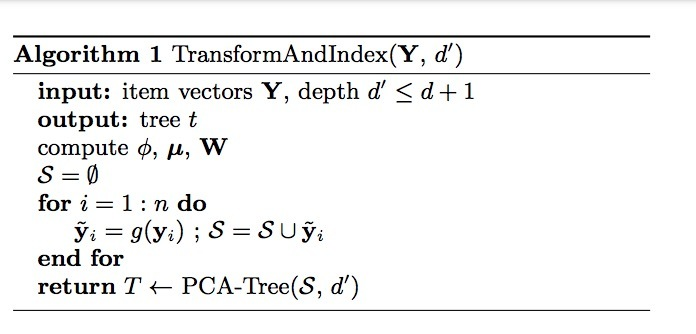# xbox IP-2-Euclidean转换介绍

## February 20, 2019

microsoft在开放了inner product快速计算的方法:《Speeding Up the Xbox Recommender System Using a Euclidean Transformation for Inner-Product Spaces》。主要解决inner product top-k search问题，我们来看下：

# 介绍

• (1) item目录(catalog)
• (2) users
• (3) 用户反馈(ratings)

• 第一部分：学习阶段，基于user feedback的离线模型学习
• 第二部分：检索阶段，对每个用户(在线)推荐items

MF是CF中最流行的方法。该方法要比其它近邻方法要好。在MF模型中，users和items通过latent feature vectors表示。Bayesian MF模型是Xbox推荐系统的核心，它每天会为数百万的用户提供游戏、电影、音乐推荐服务。在该系统中，users和items通过$R^{50}$的低维向量表示。用户u通过向量$x_u$表示，item i通过$y_i$表示，它们间的匹配质量(match quaity)通过两个向量间的内积$x_u \cdot y_i$来表示。内积越高表示该用户越愿意消费该item。

Xbox的catalog包含了上百万的items。如果使用线性扫描，每个推荐都需要数百万内积计算。user vectors会吸收上下文信息，这些信息只在用户有行为时（engagement）提供。因而，user vector的计算是实时(online)的。结果是，推荐的items列表的检索只能在线(online)执行，不能离线预计算。该任务构成了在online servers引入的单个最大密集计算任务。因此，该过程需要有个快速的替代方案。

• 小写字母表示scalars
• 粗体小写字母表示vector
• 粗体大写字母表示matrix

• $x_i$表示在维度i上的measure，具有：$(x_1, x_2, \cdots, x_d)^T \in R^d$
• norm通过$\| \cdot \|$来表示；欧氏空间中，$\|x\|=\sqrt{\sum\limits_{i=1}^d x_i^2}$。
• 我们通过$x \cdot y$来表示x和y间的内积dot product (inner product)。
• 最终，我们使用$(a, x^T)^T$来表示一个标量a与一个向量x进行拼接。

# 3.简化搜索问题（REDUCIBLE SEARCH PROBLEMS）

$s : I \times Q \rightarrow \lbrace 1,2, \cdots, n \rbrace$

$j = s_1(I,q) \ 当且仅当 j=s_2(g(I), h(q))$

• MIP：在$R^d$中的n个vectors上的最大内积(maximum inner product)。为$MIP_{n,d}$
• NN：在$R^d$中n个vectors的最近邻(nearest neighbor)，为($NN_{n,d}$)
• MCS：在$R^d$中n个向量的最大cosine相似度。($MCS_{n,d}$)

\begin{align} s(Y,x) &= argmax_i (x \cdot y_i) && MIP_{n,d} \\ s(Y,x) &= argmin_i \| x - y_i \| && NN_{n,d} \\ s(Y,x) &= argmax_i \frac{x \cdot y_i}{\| x\| \| y_i \|} && MCS_{n,d} \end{align}

• $MCS_{n,d} \leq_{O(n)} MIP_{n,d} \leq_{O(n)} NN_{n,d+1}$
• $NN_{n,d} \leq_{O(n)} MCS_{n,d+1} \leq_{O(n)} MIP_{n,d+1}$

## 3.1 保序转换(Order Preserving Transformations)

$MIP_{n,d} \leq_{O(n)} NN_{n,d+1}$

\begin{align} & \| \hat{x} \|^2 = \| x \|^2 \\ & \|\hat{y}_i \|^2 = \phi^2 - \| y_i ||^2 + \|y_i\|^2 = \phi^2 \\ & \hat{x} \cdot \hat{y}_i = \sqrt{\phi^2 - \| x_i \|^2} \cdot 0 + x \cdot y_i = x \cdot y_i \end{align}

$\| \hat{x} - \hat{y} \|^2 = \|\hat{x} \|^2 + \|\hat{y} \|^2 - 2 \hat{x} \cdot \hat{y}_i = \|x\|^2 + \phi^2 - 2x \cdot y_i$

$j = argmin_i || \hat{x} - \hat{y}_i ||^2 = argmax_i (x \cdot y_i)$

$NN_{n,d} \leq_{O(n)} MIP_{n,d+1}$

$j = \underset{i}{argmax} \ \hat{x} \cdot \hat{y}_i = \underset{i}{argmin} (\|x\|^2 + \|y_i \|^2 - 2x \cdot y_i) \\ = \underset{i}{argmin} \ \|x - y_i \|^2$

MIP搜索可以被嵌入到一个MCS search中，通过增加1维来实现：

$MIP_{n,d} \leq_{O(n)} MCS_{n,d+1}$

$\phi \triangleq max_i \|y_i \|$，假设：$\hat{y}_i = g(y_i) = (\sqrt{\phi^2 - \|y_i\|^2}, y_i^T)^T$。

$\hat{x} = h(x)= (0, x^T)^T$

$j = \underset{i}{argmax} \frac{\hat{x} \cdot \hat{y}_i}{\| \hat{x} \| \|\hat{y}_i \|} = \underset{i}{argmax} \frac{x \cdot y_i}{\| x \| \phi} = \underset{i}{argmax} x \cdot y_i$

$MCS_{n,d} \leq_{O(n)} MIP_{n,d}$

$j = argmax_i \hat{x} \cdot \hat{y}_i = argmax_i \frac{x \cdot y_i}{\|x \| \|y_i \|}$

$NN_{n,d} \leq_{O(n)} MCS_{n,d+1}$

$j = argmax_i \frac{\hat{x} \cdot \hat{y}_i}{ \|\hat{x}\| \|\hat{y}_i \|} = argmax_i \frac{x \cdot y_i}{\|x\| \phi} = argmax_i x \cdot y_i = argmin_i \|\hat{x} - \hat{y}_i \|^2$

# 4.我们的方法

• 1.将问题简化到一个Euclidian search problem
• 2.使用一个PCA-Tree来求解它。

## 4.1 简化

\begin{align} y_i^* &= g_1(y_i) = ( \sqrt{\phi^2 - \|y_i \|^2}, y_i^T)^T \\ x^* &= h_1(x)=(0, x^T)^T \end{align}

…(2)

$(Y^* - M) = W \Sigma U^T$

\begin{align} \hat{y}_i = g_2(y_i^*) = W^T (y_i^* - \mu) \\ \hat{x} = h_2(x^*) = W^T (x^* - \mu) \end{align}

…(3)

$g(y_i) = g_2(g_1(y_i)), h(x) = h_2(h_1(x))$

…(4)# 参考

Updated on

### DTS介绍

youku在《Deep Time-Stream Framework for Click-Through Rate Prediction by TrackingInterest Evolution》提出了一个兴趣演进的框架。# 摘要CTR预测在像视频推荐等工业应用中是一个必要...… Continue reading

#### taobao Privileged Features Distillation介绍

Published on December 16, 2020

#### DFN介绍

Published on December 15, 2020